## Heat Transfer Miscellaneous

#### Heat-Transfer

1. Consider one dimensional steady state heat conduction across a wall (as shown in figure below) of thickness 30 mm and thermal conductivity 15 W/mK. At x = 0, a constant heat flux, q'' = 1 × 105 W/m2 is applied. On the other side of the wall, heat is removed from the wall by convection with a fluid at 25°C and heat transfer coefficient of 250 W/m2K. The temperature (in °C), at x = 0 is _______.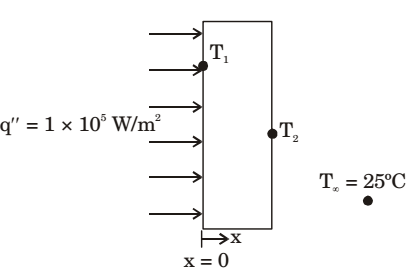1.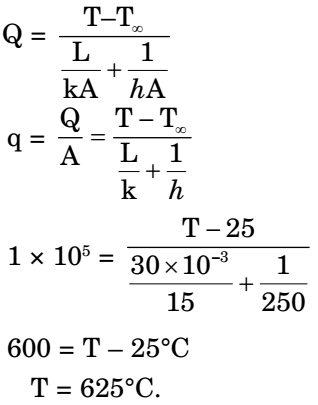##### Correct Option: A1. Consider one-dimensional steady state heat conducting along x-axis (0 ≤ x ≤ L), through a plane wall with the boundary surfaces (x = 0 and x = L) maintained at temperature of 0°C and 100°C. Heat is generated uniformly through out the wall. Choose the CORRECT statement

1.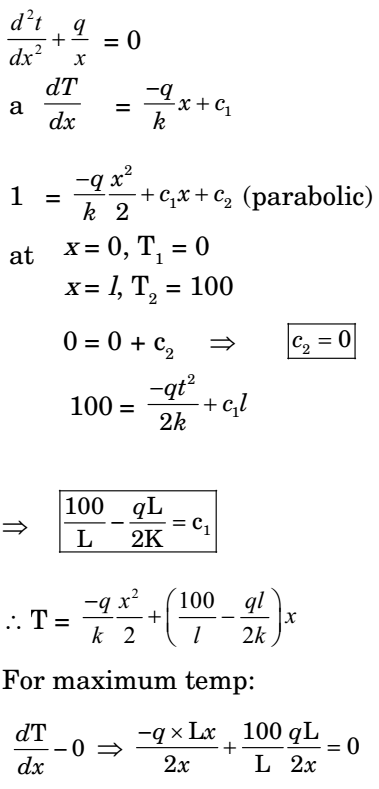##### Correct Option: B1. Consider one-dimensional steady state heat conduction, without heat generation, in a plane wall; with boundary conditions as shown in the figure below. The conductivity of the wall is given by k = k0 + bT; where k0 and b are positive constant, and T is temperature.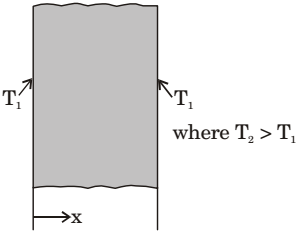As x increases, the temperature gradient (dT/dx) will

1.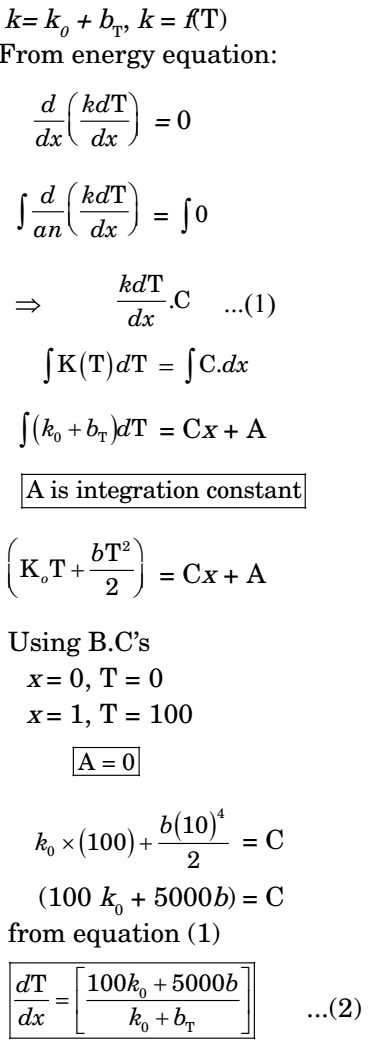##### Correct Option: A1. A thin vertical flat plate of height L, and infinite width perpendicular to the plane of the figure, is losing heat t o t he sur r oundi ngs by nat ur al convection. The temperature of the plate and the surroundings, and the proper ties of t he surrounding fluid, are constant. The relationship bet ween t he average Nussel t and Rayl ei gh numbers is given as Nu = K Ra1/4, where K is a constant. The lengt h scales for Nusselt and Rayleigh numbers are the height of the plate. The height of the plate is increased to 16L keeping all other factors constant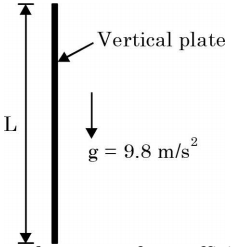If the average heat transfer coefficient for the first plate is h1 and that for the second plate is h2, the value of the ratio h1 /h2 is

1.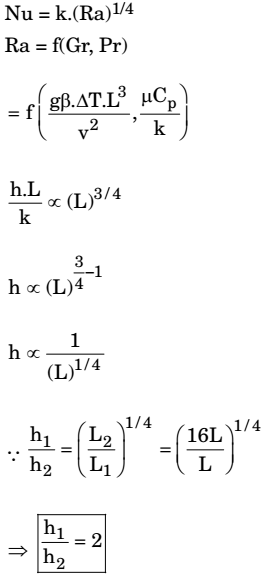##### Correct Option: A1.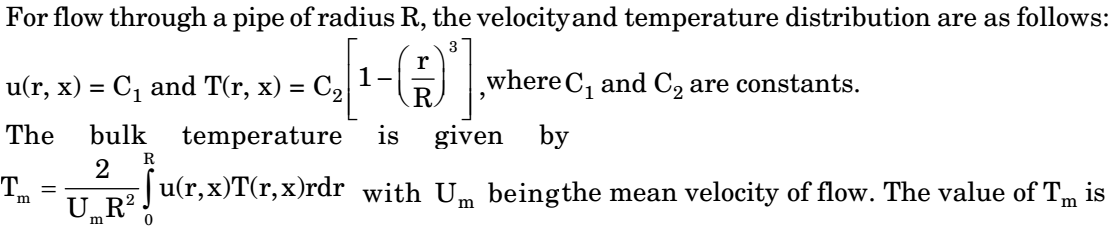1.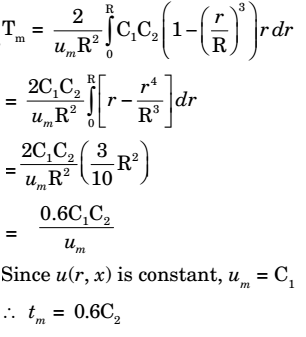##### Correct Option: C